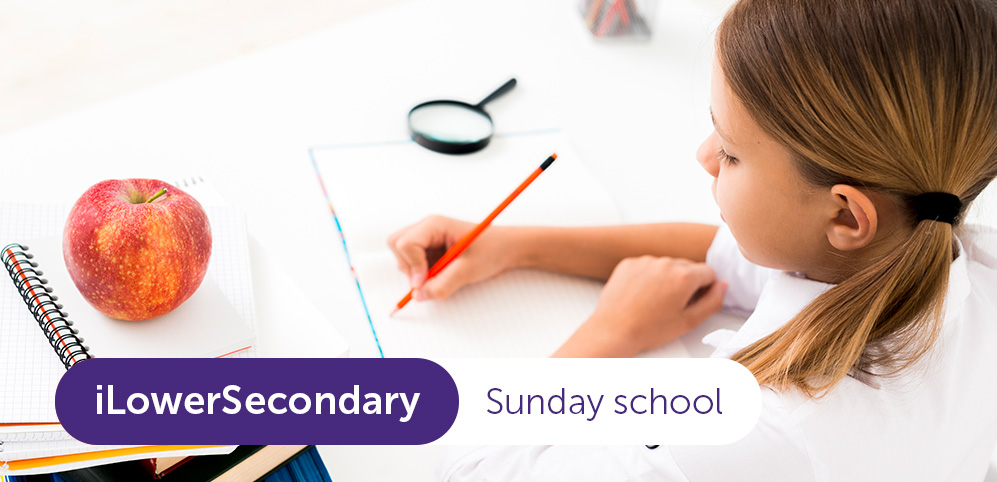Designed and built with care, filled with creative elements

Top

## iLowerSecondary | Mathematics

Categories:
€2280The main aim of iLowerSecondary Mathematics program is to ensure that key Mathematics skills are properly embedded and that students understand all the basic concepts needed to be competent in Mathematics. Developed for the non-native speakers of English, the iLowerSecondary Mathematics curriculum gives an excellent platform for later learning,  promotes engagement and enjoyment and ensures students are well prepared for their International GCSEs.

### What would students know as a result of completing this course?

After studying on this course students will be able to:

• Become fluent in the fundamentals of mathematics.
• To recall and apply complex formulae, terminology and facts with increasing speed and accuracy.
• Develop conceptual understanding.
• Apply detailed understanding of complex mathematical procedures to a given context.
• Present and organise data in a variety of forms with limited guidance.
• Interpret information from graphs, tables, charts and lists and draw conclusions.
• Use a logical approach to generate efficient strategies to solve problems by translating problems into a series of mathematical processes.
• Solve problems by applying their mathematics to a variety of routine problems, including breaking down problems into a series of simpler steps.
###### What level of English do students need to study Maths in English?

To start the course of iLowSecondary Maths students need to have a B1 (Intermediate) level of English. At this level students:

• Are able to understand the main points of clear texts in standard language if they are about topics with which they are familiar.
• Is able to produce simple, coherent texts about topics with which they are familiar or in which they have a personal interest.
• Can describe experiences, events, wishes and aspirations, as well as briefly justifying opinions or explaining plans.

#### Topics Skills

Topics:

• Properties of numbers
• Algebra
• Geometry
• Fractions and percentages
• Probability theory
• Statistics

##### Skills
• Understand and use mathematical vocabulary.
• Operate with confidence the system of numbers, including fractions, degrees and roots; make and solve algebraic expressions, equations, proportions.
• Calculate and display function graphs.
• Develop an understanding of mathematical principles.
• Analyse and solve problems, present the solutions clearly, and check and interpret the results, find the most effective way to solve the problem, select arguments when solving multistage problems.
• Make drawings and sketches of familiar objects, make prediction basing on rules of probability.
• Develop the ability to reason logically, make deductions and inferences, and draw conclusions.
• Interpret statistical tables.
• Recognise when and how a situation may be represented mathematically, apply mathematics in everyday situations.
• Acquire a foundation for further study of mathematics or for other disciplines.